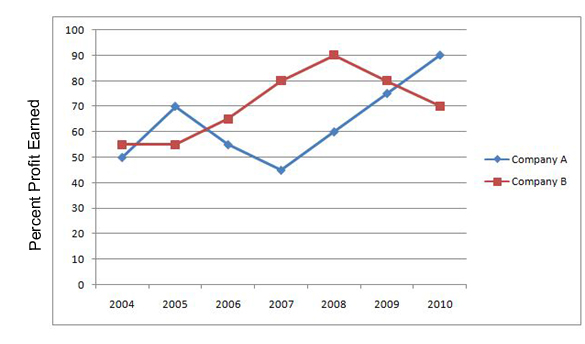## IBPS Clerk Data Analysis Test 4

Instructions

Study the following graph carefully to answer the questions.
Percent Profit Earned by Two Companies Producing Electronic Goods over the Years
percent profit =$$\frac{Profit Earned}{Total Investment}\times100$$
Profit Earned = Total Income - Total Investment in the yearQ 1

If the profit earned in 2006 by Company B was Rs. 8,12,500/-. what was the total income of the Company in that year?

Q 2

If the amount invested by the two Companies in 2005 was equal, what was the ratio between total income in 2005 of the Companies A and B respectively?

Q 3

If the total amount invested by the two Companies in 2009 was Rs. 27 lakhs, while the amount invested by Company B was 50% of the amount invested by Company A, what was the total profit earned by the two Companies together?

Q 4

If the incomes of Company A in 2007 and 2008 were equal and the amount invested in 2007 was Rs. 12 lakhs, what was the amount invested in 2008?

Q 5

If the amount of profit earned by Company A in 2006 was Rs.10.15 lakhs, what was the total investment?